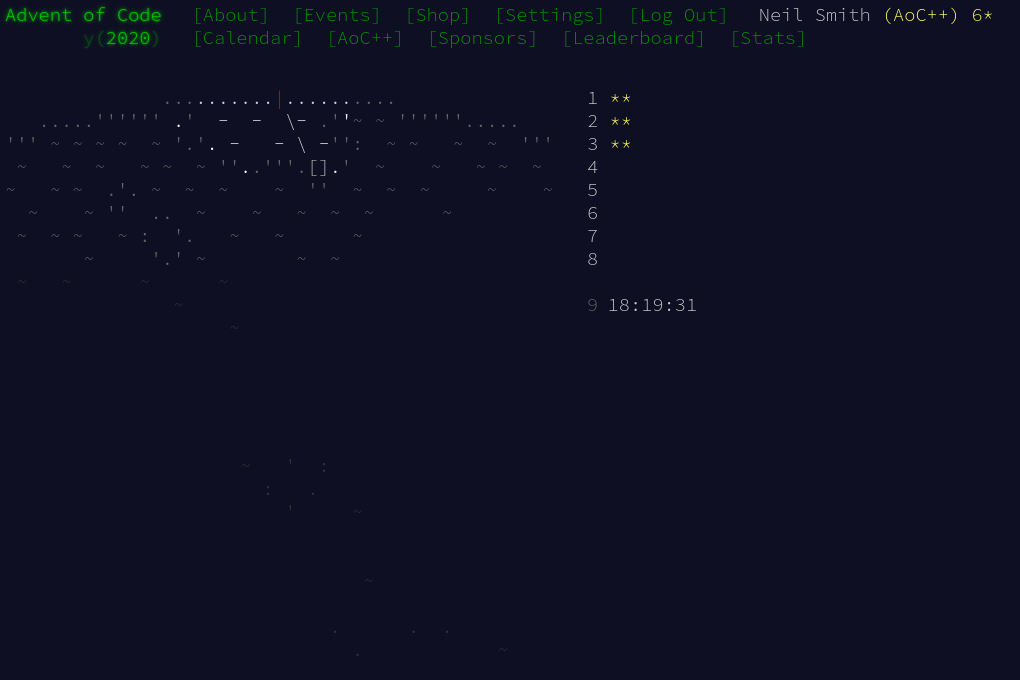December 10, 2020

# Advent of Code 2020 day 3

Sledding and lazinessDay 3 showed both the advantages of lazy evaluation, and the constant pain of off-by-one errors.

My first decision was how to represent the grid of trees. I decided on a Set of points, one for each tree. The next decision was how to describe each point: as (x, y) coordinates, or as (row, column) coordinates? I decided on (row, column) for no particular reason. I did think about representing each position as a complex number, but I quickly discovered I never used both parts together in the same way.

Reading the grid was essentially zipping the elements of the grid with their indices, and keeping the indices that contain a tree.

readGrid input = (trees, (maxR, maxC))
where trees = S.fromList $concat [ [(r, c) | (t, c) <- zip row [0..], t == '#'] | (row, r) <- zip rows [0..] ] rows = lines input maxC = (length$ head rows) - 1
maxR = (length rows) - 1


Given a set of tree locations, I then had to generate a set of locations visited by the sled. The size of the intersection of these sets would give the number of trees visited. I could either wrap the map (using cycle) or wrap the indices (using modulus). Given I already had a set rather than separate rows, I decided to wrap the indices.

The places visited by the sled are generated as an infinite list of positions (using iterate, but taking only those within the grid (using takeWhile withinTrees ).

part1 trees maxCorner = countEncounteredTrees trees maxCorner (1, 3)

countEncounteredTrees trees maxCorner delta = S.size $S.intersection trees visited where visited = S.fromList$ takeWhile (withinTrees maxCorner) $visitedPlaces delta maxCorner visitedPlaces :: Position -> Position -> [Position] visitedPlaces (dr, dc) (_maxR, maxC) = iterate wrappingStep (0, 0) where wrappingStep (r, c) = (r + dr, (c + dc) mod (maxC + 1)) withinTrees (maxR, maxC) (r, c) = r >= 0 && r <= maxR && c >= 0 && c <= maxC  Part 2 was much the same, but using several steps and multiplying their results together with product. part2 trees maxCorner = product$ map cet [(1, 1), (1, 3), (1, 5), (1, 7), (2, 1)]
where cet = countEncounteredTrees trees maxCorner


## Code

You can find the code here or on Gitlab.